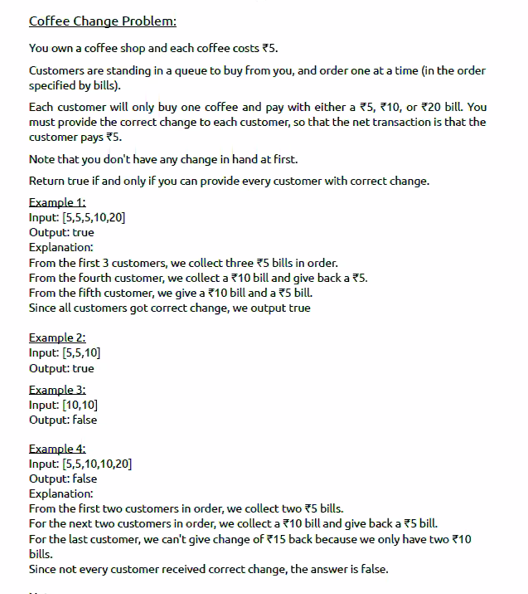# Oracle Coding Question: Coffee Change Problem

This programming question was asked by Oracle recently. We recommend you to try it out on your own first. Then verify with the answer.## Solution:

```public class Solution {

public static void main(String[] args) {
Integer[] input = {
5,
10,
10,
20
};
int noOfFives = 0;
int noOFTens = 0;
boolean output = true;
if (input == 10 || input == 20) {
output = false;
}

for (int i = 0; i < input.length; i++) {
if (input[i] == 5) {
noOfFives++;
} else if (input[i] == 10) {
noOFTens++;
if (noOfFives > 0) {
noOfFives = noOfFives - 1;
} else {
output = false;
}

} else if (input[i] == 20) {

if (noOFTens > 0 && noOfFives > 0) {
noOfFives = noOfFives - 1;
noOFTens = noOFTens - 1;
} else if (noOfFives > 3) {
noOfFives = noOfFives - 3;
} else {
output = false;
}
}

}

System.out.println(output);
}
}```

Hope you found it easy, It can be solved using dynamic programming also. But we leave it for you. If you can crack it the you are welcome to comment below. If solution is good then we might add here in this article.